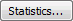# Geometry Statistics

For a Probabilistic analysis you can define the following slope geometry parameters as random variables in the Input Data dialog:

• Slope Angle
• Slope Height
• Upper Slope Angle
• Toppling Joints Spacing
• Toppling Joints Dip
• Overall Base Inclination
• Rock Unit Weight

For details of the slope geometry parameters, see Slope Geometry Input in RocTopple.

To define an input parameter as a random variable, select a statistical distribution for the variable (e.g., Normal, Uniform, etc.) and enter the required statistical parameters (e.g., mean, standard deviation, minimum and maximum values).

• Click the Quick-Stats buttonnext to the variable in the Input Data Geometry tab.
A popup dialog appears allowing you to select a Distribution and enter the standard deviation, relative minimum, and relative maximum. This option is handy for quickly defining statistics for individual variables.
For details on defining statistical distributions, standard deviation, and minimum/maximum values, see Overview of Statistical Distributions in RocTopple.After you have selected a distribution, the button changes to indicate the type of distribution selected (e.g., for a Normal Distribution, the button will show as). In addition, mousing over the button displays a popup showing the input.

NOTE: When defining Geometry and Strength variables, you also have the option of using the Statistics buttonat the bottom of the Input Data dialog to define multiple random variables at a time.